## php源码学习d6 字符串2

1.柔性数组

``````struct _zend_string {
zend_refcounted_h gc; /* 垃圾回收 */
zend_ulong h; /* hash value */
size_t len; // 存长度，内存占用8
char val; // 柔性数组 比char* 指针的好处，地址连续，从内存取一次即可
};``````

char val[] 不占内存

char var 占内存

char var

PHP中的字符串的结构决定字符串变量申请内存时是连着后边字符实际所占内容一块申请，即

lenth(_zend_string)+len+1

2.redis

3.写时复制

``````\$a = 10;
echo \$a;
\$b = \$a;  // 直接复制
echo \$b;``````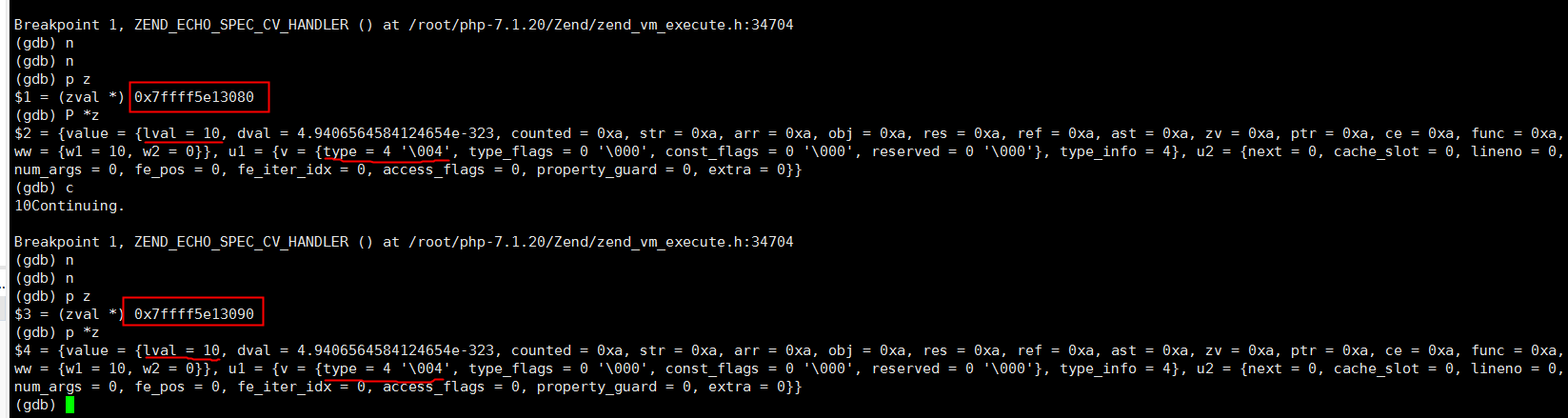``````\$a = 'sting'.date('y-m-d');
echo \$a;
\$b = \$a;  //不复制 变量的地址不同，指向的字符串地址一样
echo \$b;
\$b = 'abcd'; // 复制， a,b指向的字符串地址变了
echo \$a;
echo \$b;``````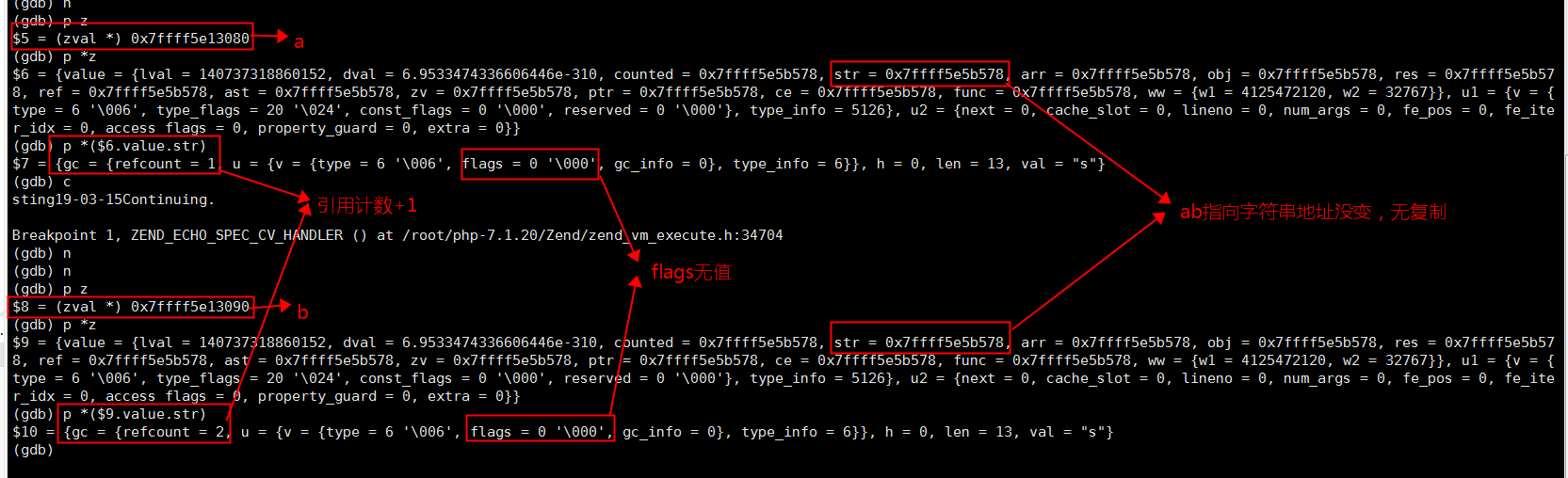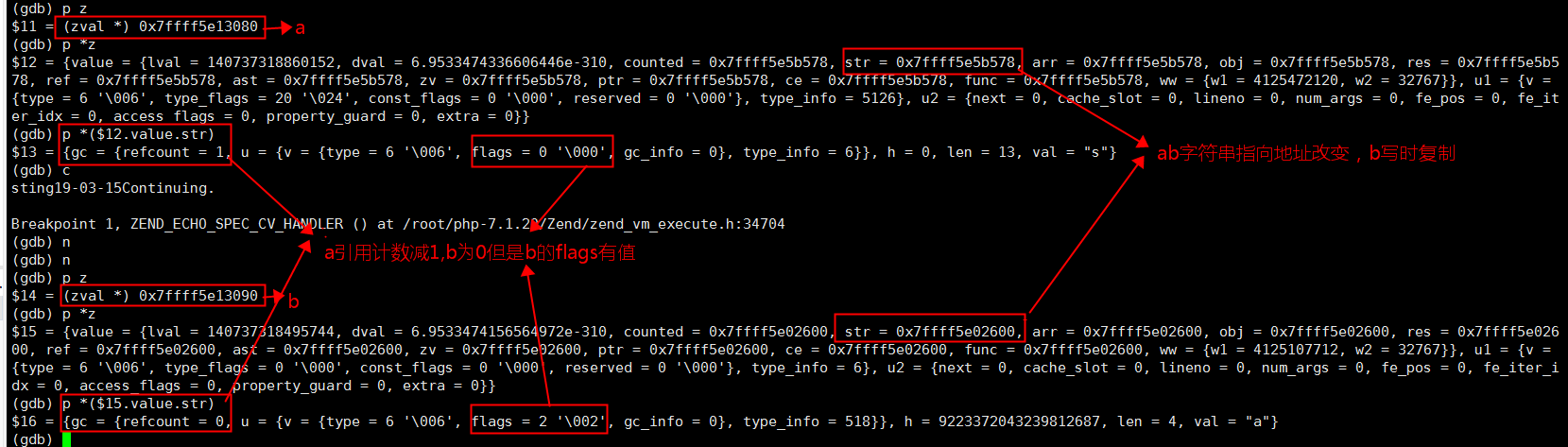``````\$a = 'string';
echo \$a;
\$b = \$a;  //不复制 变量的地址不同，指向的字符串地址一样
echo \$b;
\$b = 'abcd'; // 复制， a,b指向的字符串地址变了，自变量flags标识，不用refcount 区别于date() 变量
echo \$a;
echo \$b;``````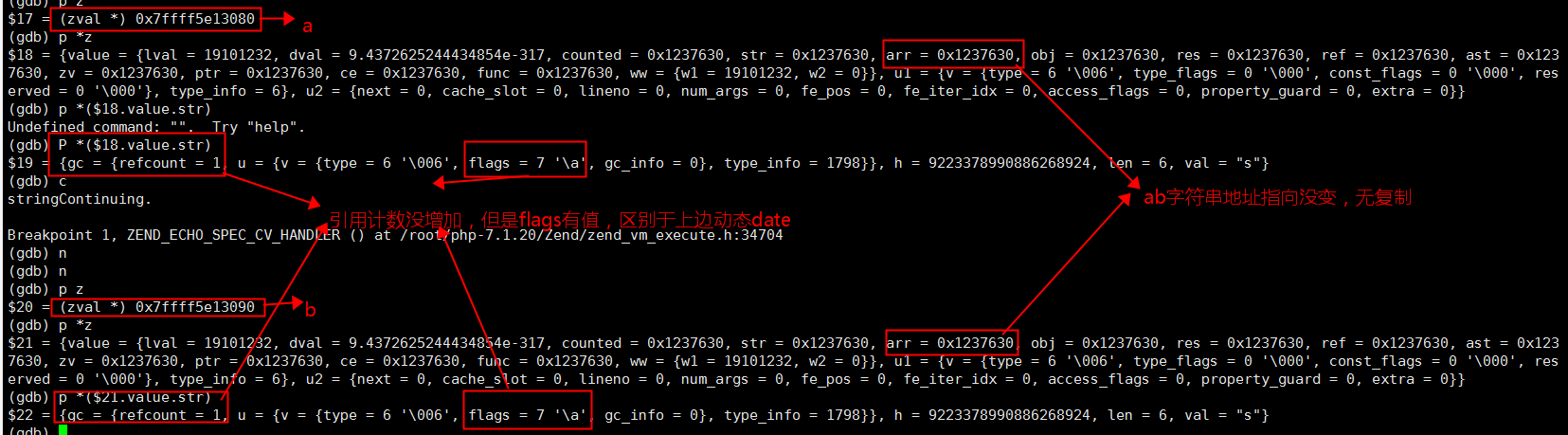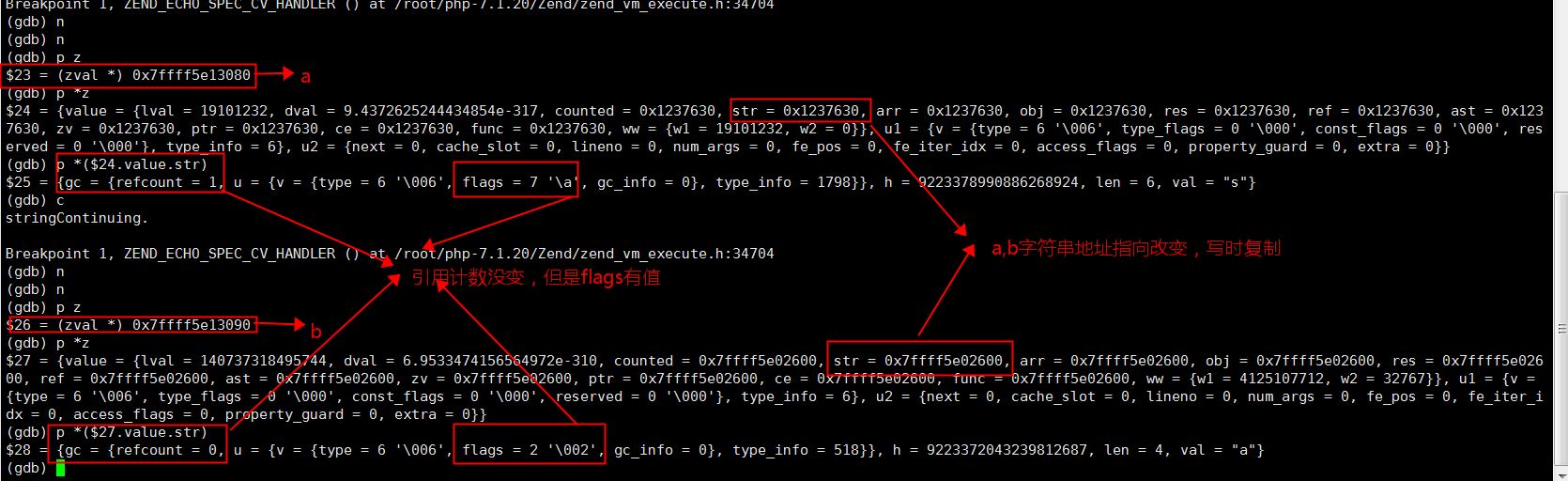4.符号表

php7中有一个符号表（hashtable类型）来存储变量名字, val是变量，key是变量的地址。\$_GET等7个全局变量也存里边，如下图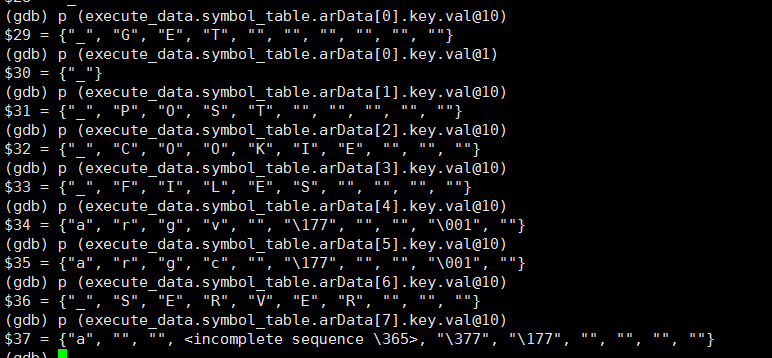5.引用

``````\$a  = 1;
echo \$a; // type = 4 IS_LONG 整型
\$b = &\$a;
echo \$a；// type = 10 IS_REFERENCE 引用
echo \$b; // type = 10 IS_REFERENCE 引用
unset(\$b)
echo \$b; // type = 0 IS_UNDEF undefined
\$c =\$a;
echo \$a; // 10 地址改变
echo \$c; // type = 4 IS_LONG 整型
\$a = 1;
\$b = &a; // type = 10 IS_REFERENCE 引用
\$c = &b; // type = 10 IS_REFERENCE 引用,不是引用的应用``````
`` ``

6.\0 是一个字符

7.扩展

``````// ab公用，不会存两份
\$a = 'abcd';
echo \$a;
\$b = 'abcd';
echo \$b;``````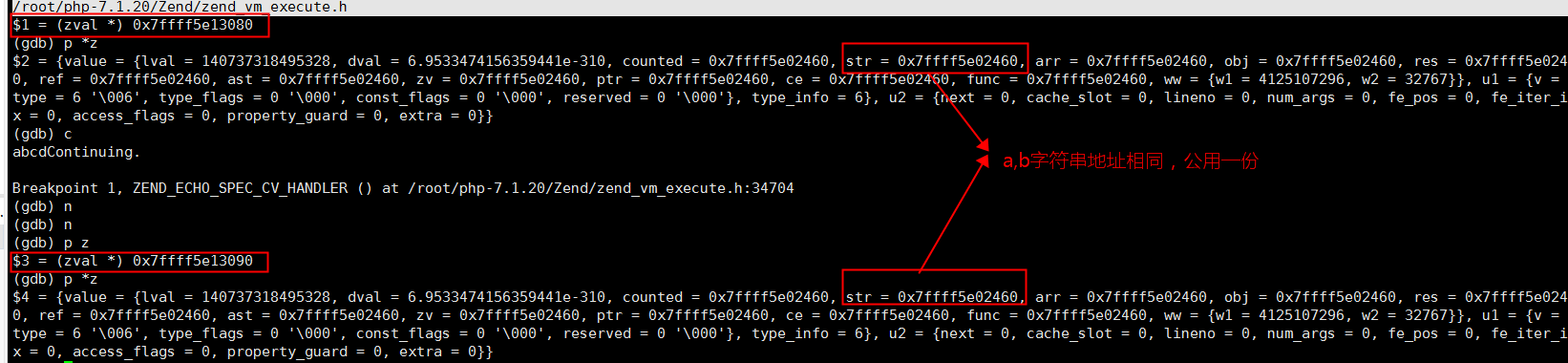8.笔记地址

d6 字符串2

0条评论